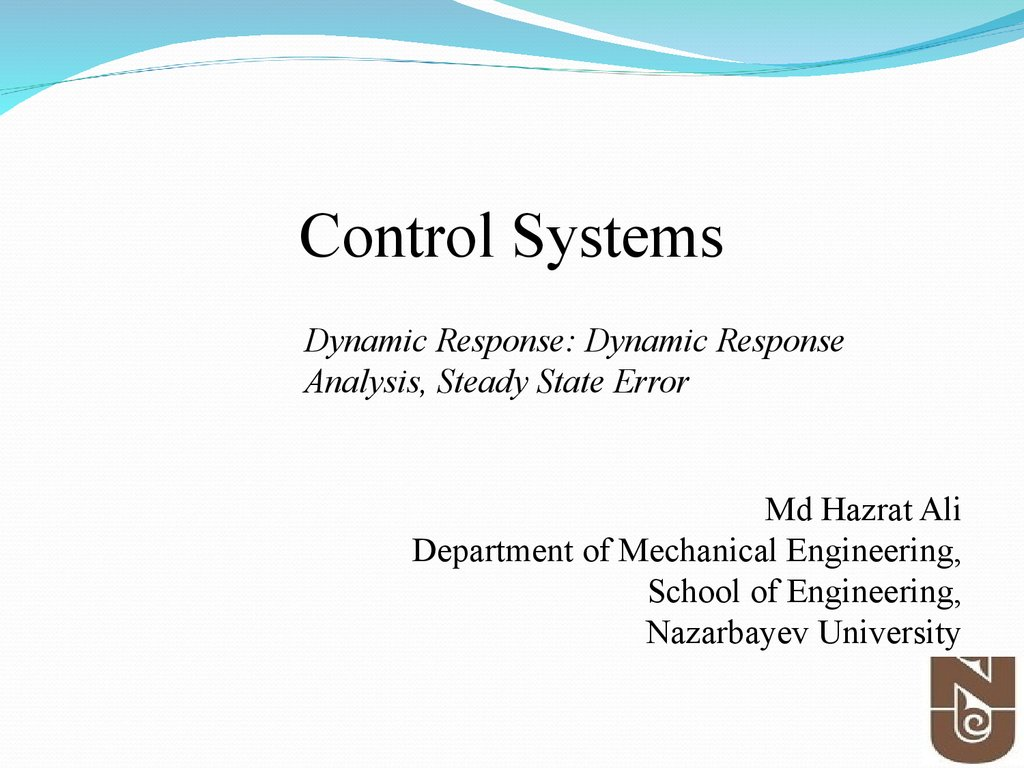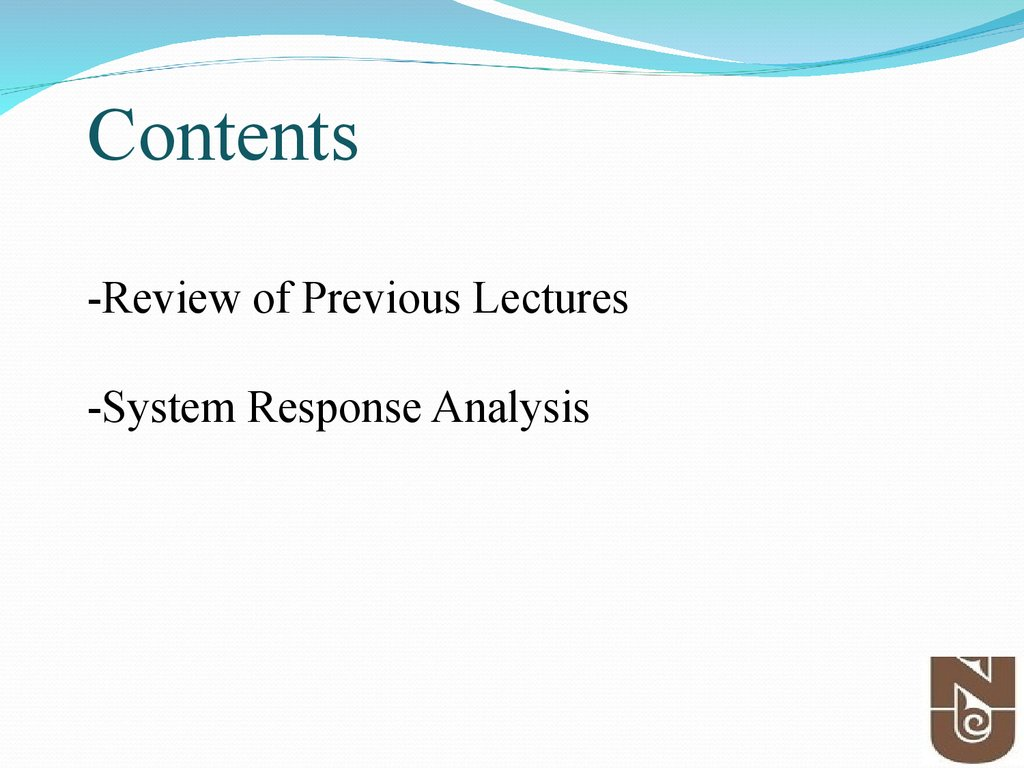# Control systems

## 1.

Control Systems
Dynamic Response: Dynamic Response
Analysis, Steady State Error
Md Hazrat Ali
Department of Mechanical Engineering,
School of Engineering,
Nazarbayev University

## 2.

By failing to prepare, you are
preparing to fail.
Benjamin Franklin

## 3.

Contents
-Review of Previous Lectures
-System Response Analysis

## 4. Review

• Once transfer function is obtained, we can start
to analyze the response of the system it
represents .
• A block diagram is a convenient tool to visualize
the systems as a collection of interrelated
subsystems that emphasize the relationships
among the system variables.
• Signal flow graph and Mason’s gain formula are
used to determine the transfer function of the
complex block diagram.

## 5. Review-Block Diagram

• Three Elementary Block Diagrams
– Series connection
– Parallel connection
– Negative Feedback connection

## 6. Negative feedback :Single-loop gain

The gain of a single-loop negative feedback system is
given by the forward gain divided by the sum of 1 plus the
loop gain.
Franklin et.al- pp.122

## 8. Table 2.6 (continued) Block Diagram Transformations

Review-Block Diagram
Table 2.6 (continued) Block Diagram Transformations

## 9. Review-Block Diagram

Practice: Find the transfer function of the
following block diagram
Y(s)
2s 4
2
R (s) s 2s 4

Practice:

## 12.

Time domain and frequency domain

## 17. Poles and Zeros

H (s) K
m
i 1
n
i 1
( s zi )
( s pi )
K is the transfer gain
The roots of numerator is called zeros of the system. Zeros
correspond to signal transmission-blocking properties.
The roots of denominator are called poles of the system. Poles
determine the stability properties and natural or unforced
behavior of the system.
Poles and zeros can be complex quantities.
zi=pi, cancellation of pole-zero may lead to undesirable system
properties.

## 20.

Key points: Effect of Poles and Zeros

## 21. System Response

Example:
Consider the following transfer function
Y (s)
2s 1
2
R ( s ) s 3s 2
Determine:
• Poles and Zeros?
21

## 23. System Response

Example:
Consider the following transfer function
Y ( s)
s 3
2
R ( s ) s 5s 6
Determine:
• Poles and Zeros?
23

## 24. Time-Domain Specification

Test Input Signals
• To measure the performance of a system we use
standard test input signals. This allows us to compare
the performance of our system for different designs.
• The standard test inputs used are the step input, the
ramp input, and the parabolic input.
• A unit impulse function is also useful for test signal
purpose.

## 25. Time-Domain Specification

Test Input Signals
step input
ramp input
parabolic input
A, t 0
r (t )
0, t 0
A
R( s)
s
At , t 0
r (t )
0, t 0
A
R( s) 2
s
At 2 , t 0
r (t )
0, t 0
2A
R( s) 3
s
The step input is the easiest to generate and evaluate and is
usually chosen for performance tests.

## 26. Time-Domain Specification

Example
The transfer function:
9
G (s)
s 10
The system response to a unit step input (A=1):
y (t ) 0.9 1 e 10t
y ( ) 0.9

## 27. System Response

Example 2:
Consider the following transfer function
Y ( s)
7s 1/ 2
2
R ( s ) s 5s 6
Determine:
• impulse response

## 28.

First Order System Response

## 29.

First Order System Response
Example 1:
Consider the following transfer function
Y (s)
1
H (s)
R(s) s
Determine:
• impulse response(response when r(t) is
impulse function)
•Classify stability

## 30.

First Order System Response- Impulse response
1/

## 31.

Standard Second Order System
• Let us consider the following closed-loop system:
• The TF of the closed-loop system:
G(s)
K
T ( s)
2
1 G ( s ) s ps K
• Utilizing the general notation of 2nd Order System:
T (s) 2
s 2 n s n2
2
n
• Where n is natural frequency and is damping ratio

## 32.

Standard Second Order System
d n 1
2
cos 1
0 1

## 33. Figure 3.24 Graphs of regions in the s-plane delineated by certain transient requirements: (a) rise time; (b) overshoot; (c) settling time; (d) composite of all three requirements

Transformation of the specification to the splane
Figure 3.24 Graphs of regions in the s-plane delineated by certain transient requirements: (a) rise time; (b) overshoot;
(c) settling time; (d) composite of all three requirements

## 34. Transformation of the specification to the s-plane

Example 3.25
Find allowable regions in the s-plane for the poles
transfer function of system if the system response
requirements are tr ≤ 0.6, Mp <= 10% and ts <= 3 sec.

## 35.

Standard Second Order System
Mp?

## 36.

Standard Second Order System
Standard Second Order System
Poles (roots) location of the second order complex
system.
s1,2 n j 1 2 n n j d
where
d is the "damped natural frequency".

## 37.

Standard Second Order System
• Classification of Type Response of 2nd Order Systems
Undamped: =0
Critical damped: =1
Under-damped: 0< <1
Over-damped: >1

## 38.

Standard Second Order System
As decreases, the response becomes increasingly
oscillatory.

## 39. Time-Domain Specification

• Standard performance measures are usually defined in term
of the step response of a 2nd order systems:

## 40. Time-Domain Specification

• Standard performance measures are usually defined
in term of the step response of a 2nd order systems:
– Rise time, Tr : time needed from 0 to 100% of fv for
underdamped systems and Tr1 from 10­90% of fv for
overdamped systems.
– The settling time ts is the time it takes the system transient
to decay.
– The overshoot Mp is the maximum amount of the system
overshoots its final value divided by its final value.
– The peak time tp, is the time it takes the system to reach
the maximum overshoot.

## 41. Time-Domain Specification

-Rise Time, Tr-
A precise analytical relationship between rise time
and damping ratio cannot be found. However, it
can be found using numerically using computer.
A rough estimation of the rise time is as follows
1.8
tr
n

## 42. Time-Domain Specification

-Maximum Overshoot, Mp
Maximum overshoot (in percentage) is defined as
Mp
' Peak Value ' ' Final Value '
100%
' Final Value '
M p 100e
1 2

## 43. Time-Domain Specification

-Peak Time TpTp is found by differentiating y(t) and finding the
first zero crossing after t=0.
Tp
d n 1 2

## 44. Time-Domain Specification

-Settling Time Ts-
For a second order system, we seek to determine the
time Ts for which the response remains within
certain percentage (1%, 2% ) of the final value.
For 1% settling time
4.6.6
4
Ts
T
s
n n
For 2% settling time
4
Ts
n

## 45. Time-Domain Specification

Exercise # 1
Find Tr, Tp, Mp and Ts for the following transfer
function:
25
T (s) 2
s 30 s 225
45

## 46. Time-Domain Specification

Exercise # 2
Find Tr, Tp, Mp and Ts for the following transfer
function:
19
T (s) 2
s 24 s 19 / 3
46

## 47. Exercise # 3

If the system response requirements are tr = 0.6, Mp =
10% and ts = 3 sec.
Find:
n ,
47

## 48. Exercise # 4

Problem# If the system response requirements are tr =
0.6, Mp = 10% and ts = 3 sec.
Find:
n ,
For 1% settling time
1.8
tr
n
4.6
Ts
n
48

## 49. Time-Domain Specification

Exercise # 5
Find Tr, Tp, Mp and Ts for the following transfer
function:
1
T (s) 2
s 15s 100

## 50. Time-Domain Specification

Exercise # 6
Find Tr, Tp, Mp and Ts for the following transfer
function:
5
T (s) 2
s 30 s 225
50

## 51. Time-Domain Specification

Exercise # 7
Find Tr, Tp, Mp and Ts for the following transfer
function:
3
T (s) 2
s 24 s 9
51

## 52. Figure - Multiple-loop feedback control system.

Example - Block diagram
Find TF from the given block diagram
Figure - Multiple-loop feedback control system.

## 53. Figure 2.27 Block diagram reduction of the system of Figure 2.26.

Quiz # 4- Answer to Q1
Figure 2.27 Block diagram reduction of the system of Figure 2.26.

## 54. System Response

Find TF from the given block diagram
Consider the following transfer function
Y (s)
2s 1
H (s)
2
R ( s ) s 3s 2
Determine:
i) Impulse response graphically
ii) Classify stability

## 56. Midterm Exam

March 4, 2016, Friday,
Time:8.00-9.00
Venue-6.141 & 5.103
Topics- Cover Until February

## 58. Tell me, I will forget! Show me, I may remember! Involve me, I will understand!

Benjamin Franklin

## 59. Further Reading

Franklin, et. al., Chapter 3
Section 3.1-3.6
Richard C. Dorf et.al, Chapter 3